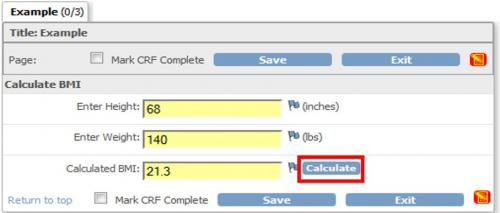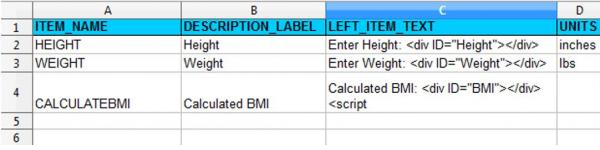One of the many useful features of OpenClinica is the ability to perform calculations within CRFs. After specifying a calculation in the CRF template, the calculation is automatically performed upon clicking save. Clicking save will either load the next section of the CRF or save the CRF and exit to the Event screen. While the current functionality will suite many needs, it may be desirable to perform the calculation prior to clicking save.

This can be accomplished using the “Calculate” button.## 12.1.1 Creating a CRF

For this example, a very simple CRF was created containing only three fields: Height, Weight, and BMI.Notice that the LEFT_ITEM_TEXT contains the text that will be visible (ie Enter Height:) along with <div ID=></div>. This script provides an identifier for each field and will be referenced by the calculation script.

## 12.1.2 The Calculation Script

A little java-script from the JQuery library will help us perform our BMI calculation. The following should be added to the LEFT_ITEM_TEXT field within your CRF Excel template:

<script src=”includes/jmesa/jquery.min.js”>// for OC versions before 3.1.4, use jquery-1.3.2.min.js !</script><script>
\$.noConflict();
{
var heightField = \$(“#Height”).parent().parent().find(“input”);
var weightField = \$(“#Weight”).parent().parent().find(“input”);
var bmiField = \$(“#BMI”).parent().parent().find(“input”);

function getBMI(height1, weight1)
{
//calculate weight portion
var weightLBS = weight1*703;
//calculate height portion
var heightINCH = height1*height1;
//divide weight by height
var bmi = (weightLBS/heightINCH);
//calculate the days
var finalBMI = Math.round(bmi*10)/10;
if (isNaN(finalBMI))
{
return 0;
}
else
{
return finalBMI;
}
}

function calcBMI()

{
//calculate the BMI given the height and weight.
var bmi = getBMI(heightField.val(), weightField.val());
//write the calculated value to the field.
if (bmiField.val() != bmi)
{
bmiField.val(bmi);
bmiField.change();
}
};
//Calculate will fire upon save or when the calculation button is clicked.
\$(“#srl”).focus(function(){ calcBMI(); });
\$(“#srh”).focus(function(){ calcBMI(); });
\$(“#calculate1”).click(function(){ calcBMI(); });
})
</script>

## 12.1.3 Adding A Fancy Button

The following script, when added to the RIGHT_ITEM_TEXT of the CRF Excel template, will insert a button to the right of the field:

<img id=”calculate1″ src=”images/Calculate.gif”>
<script src=”includes/jmesa/jquery-1.3.2.min.js”></script>
<script lang=”Javascript”>
\$.noConflict();
</script>

The button has the ID “calculate1” which is referenced in the first calculation script above:\$(“#calculate1”).click(function(){calcBMI(); });

Notice that the source of the image is images/Calculated.gif. This refers to the standard images folder of the OpenClinica interface. This example uses a custom image. To use a custom image, drop the file in the /oc/tomcat/webapps/OpenClinica/images folder. Alternatively, the OpenClinica images below already exist in the images folder:Try it yourself!